# Network Theory: An Introduction

#### Motivation

Examples of networks:

For comparison, consider this representation of the internet (Approximately 1.3 million nodes)

General questions about networks:

• What are fundamental properties of networks?

• How are biological networks different from other types of networks?

• Do properties of networks explain higher-level phenomena?

Overview of lecture:

• Study networks in an abstract sense.

• Identify and explain fundamental properties.

• Describe some aspects of an emerging "science of networks".

Exercise: What do the terms "Friendster", "Kevin Bacon" and "Six degrees" have in common?

Exercise: Use Star-links to:

• find the Bacon number of a GW-alum.
• find two names that are connected by at least three links.

Exercise: What does the term Erdos number mean?

#### Graph theory

Informal definition:

• A graph is a mathematical abstraction used to represent "connectivity information".

• A graph consists of vertices and edges that connect them, e.g.,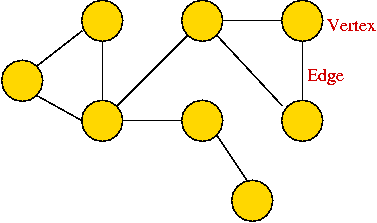• It shouldn't be confused with the "bar-chart" or "curve" type of graph.

Formally:

• A graph G = (V, E) is:
• a set of vertices V
• and a set of edges E = { (u, v): u and v are vertices }.

• Two types of graphs:
• Undirected graphs: the edges have no direction.
• Directed graphs: the edges have direction.

• Example: undirected graph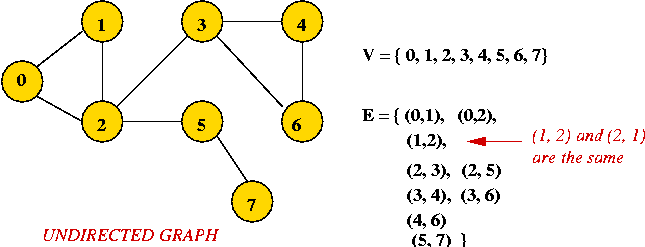• Edges have no direction.
• If an edge connects vertices 1 and 2, either convention can be used:
• No duplication: only one of (1, 2) or (2, 1) is allowed in E.
• Full duplication: both (1, 2) and (2, 1) should be in E.

• Example: directed graph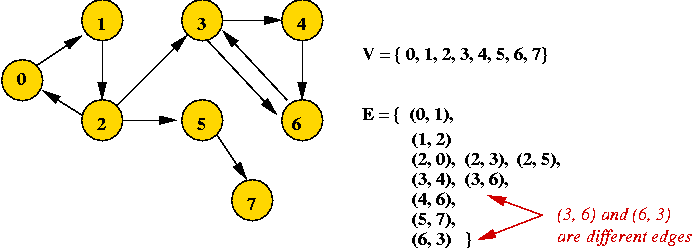• Edges have direction (shown by arrows).
• The edge (3, 6) is not the same as the edge (6, 3) (both exist above).

Depicting a graph:

• The picture with circles (vertices) and lines (edges) is only a depiction
=> a graph is purely a mathematical abstraction.

• Vertex labels:
• Can use letters, numbers or anything else.
• Convention: use integers starting from 0.
=> useful in programming, e.g. degree[i] = degree of vertex i.

• Edges can be drawn "straight" or "curved".

• The geometry of drawing has no particular meaning: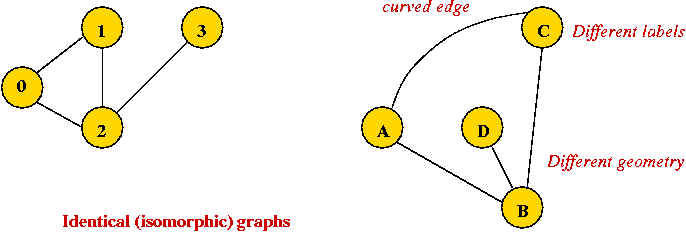Some basic definitions (for undirected graphs):

• Degree of a node the number of edges incident to it.

• Path: a sequence of vertices in which successive vertices have an edge between them.

• Connectivity: two vertices are connected if there is a path that includes them.

• Component: A subgraph is a subset of vertices together with the edges from the original graph that connects vertices in the subset.

Example: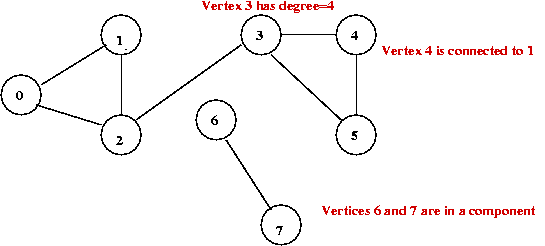Some characteristic measures:

• Degree distribution: how many nodes have degree=1, how many have degree=2, ... etc.

Consider this example: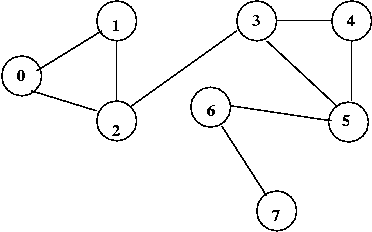In above example:  2 vertices with degree=1: 6, 7 3 vertices with degree=2: 0, 1, 4, 5 2 vertices with degree=3: 2, 3
Thus, the degree distribution is: {2, 3, 2}

• Average Shortest-Path (SP) length: compute this with the following procedure
• For each pair of vertices: compute length of shortest path connecting them.
• Take the average over all vertex-pairs.
For above example: average = 2.06

• Cluster coefficient: compute as follows
• For each vertex, compute its clustering:
• Identify neighbors.
• Identify the number of connections amongst neighbors.
• If m=#neighbors, divide clustering by (m-1)*m/2.
• Average over all vertices.
For the above example: 1.75

Exercise: Build the connectivity graph for the class. Compute the measures above.

#### The field of graph theory

History:

• Maze-searching.

• Euler's crossing problem: the Konigsberg bridges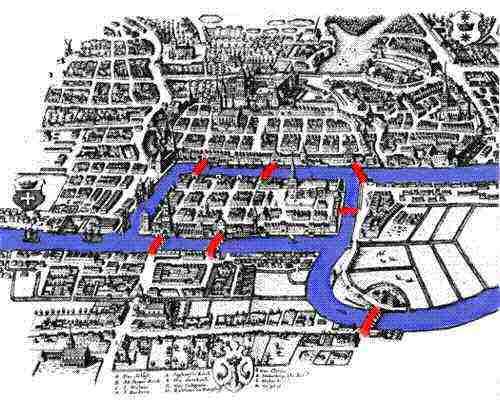How to cross each bridge just once and return to starting point?

Some famous problems in graph theory:

• Euler tour: A cycle that traverses all edges exactly once (but may repeat vertices).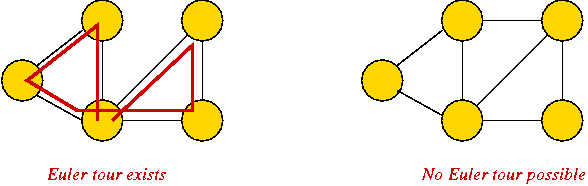Known result: Euler tour exists if and only if all vertices have even degree.

• Hamiltonian tour: A cycle that traverses all vertices exactly once.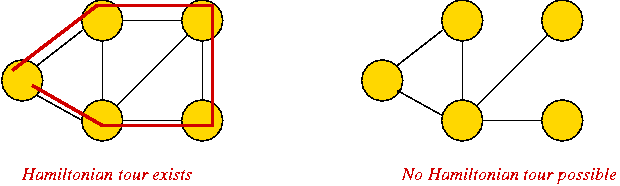Known result: testing existence of a Hamiltonian tour is (very) difficult.

• The Four-Color problem: given a map of regions, at most four colors are needed to color so that no two adjacent regions have the same color.

Exercise: How many colors are needed for the 8-node graph above?

• Traveling Salesman Problem (TSP): which tour of the vertices has the least overall length?

Applications of graph theory:

• Fundamental mathematical construct to represent "connectivity".

• Appears in thousands of problems.

• Source of many classic problems: traveling salesman, routing, spanning trees.

• Many "graph-structured" applications: networks, transportation-systems, electronic circuits, molecules.

Graph theory as a source of computer science theory:

• Many important algorithms.

• Key to understanding algorithm design and analysis.

• Simple to describe, yet perplexing:
• Euler tour: easy problem.
• Hamiltonian tour: hard problem.

The field of graph theory:

• Large area of mathematics:
• Analysis of general graphs.
• Analysis of special types of graphs.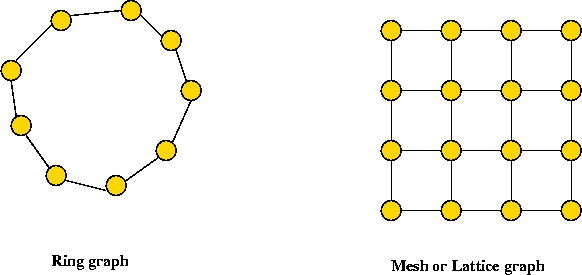• Many classic problems
e.g., the four-color theorem.
• Optimization problems based on graphs,
e.g., shortest-paths.

• Graph algorithms: an area in computer science.
• Rich source of algorithms, theory, insight.
• Useful algorithms used in many applications
(e.g., in a compiler).

#### Random graphs

Consider this procedure to generate a random graph:

• Draw n vertices.

• Consider each possible pair of vertices in turn:
• For each such pair, flip a coin.
• If heads, place an edge between these vertices.

Exercise: Split into groups of 4 or 5. Generate a random graph with 10 nodes using coin flips.

Exercise: Suppose we use a coin where Pr[heads] = 0.1. Will we get more or fewer edges than with a fair coin?

Parametrized random graphs:

• Parameter: 0 < p < 1 (density)

• Use a coin such that Pr[heads] = p to generate the graph.

Exercise: Split into groups of 4 or 5. Generate a random graph using p = 0.1.

Connectivity:

• Clearly, if p is small, we might have multiple components (disconnected graph).

• Examples (using graph tool).

• Major result (Erdos and Renyi):
• If p < 1/n, the graph is almost always disconnected.
• If p >= log(n)/n, the graph is almost always connected.
• If 1/n < p < log(n)/n, the graph is dominated by a giant component.
Other properties:
• Average path length is small: about log(n).

• Cluster coefficient is very small: less than 1.

Exercise: Can you create a graph where the average shortest-path is quite long?

#### The small world phenomenon

Small world phenomenon:

• Stanley Milgram's experiment (1967).

• Path length: For any two random persons, there exists six people to connect them.
=> short path lengths.

• Cluster coefficient: your friends are friends amongst themselves
=> high cluster coefficient.

Observation: the standard random graph does not model "small world".

The Watts-Strogatz random model:

• Two parameters:
• p: the probability of re-arrangement.
• k: the number of "ring" neighbors.

• Each node is connected to k successive neighbors.

• For each edge in turn:
• Flip a p-biased coin.
• If heads, replace edge with a random edge from one of its nodes to a random other node.

Examples: (demo)

Properties:

• For p = 0, we get a regular structure (ring-like).

• As p increases, there is more randomness.

• For p = 1, it becomes the standard Erdos-Renyi random graph.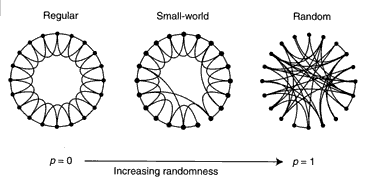(From http://www.santafe.edu/sfi/publications/Bulletins/bulletinFall99/workInProgress/smallWorld.html)

• The regular structure (ring) has high cluster coefficient, long path lengths.

• Erdos-Renyi has small path length, low cluster coefficient.

• For a range of p, you get low path length, high cluster coefficient
=> small world graph.
Examples of small world graphs.

 Lactual Lrandom Cactual Crandom Film actors 3.65 2.99 0.79 0.00027 Power grid 18.7 12.4 0.080 0.005 C. elegans 2.65 2.25 0.28 0.05 (From http://www.santafe.edu/sfi/publications/Bulletins/bulletinFall99/workInProgress/smallWorld.html)

Applications:

• (Biology) Modeling of disease epidemics.

• (Epidemiology) Efficacy of needle-exchange programs (AIDS prevention):
• Used needle = random link.
• Removal of discarded needles: reduces chances of disease percolation (epidemic).

• (Economics) Modeling of fads, momentum investing.

• (Business) Organizational hierarchy (Toyota example).

• (Commercial) meetup.com, friendster.com, tribe.com, linkedin.com

#### Power-law graphs

Exercise: Which function is "smaller" for fixed k: f(n) = e-kn or g(n) = n-k?

Consider the degree distribution of a graph:

• Let fi = number of vertices with i edges.

• One can show that for random graphs, fi is proportional to e-a*i (for some constant a).

• A power-law graph is a graph in which fi is proportional to i -k (for some constant k).
Examples:
• A random (Erdos-Renyi) example (demo).

• E-Coli gene regulation graph (demo).

• Internet, among many other real data sets.
Implications:
• Random graphs have very few high-degree nodes.

• Power-law graphs have some high-degree nodes.

• Robustness implications:
• A random removal of nodes can disconnect a random network.
• Power-law graphs are more resistant to random removals.
• Power-law graphs are extremely sensitive to targeted removals.
=> Consider what happens when you remove the high-degree nodes.

• Biological implications:
• High-degree nodes: genes involved in regulating many others (bookkeeping).
• Robustness necessary to support mutation/evolution.

#### Network motifs

A graph or network motif is a small localized sub-structure in a graph.

Notation: TF = Transcription Factor Example: Feed-Forward (FF) loop motif:

• A Feed-Forward loop has three elements: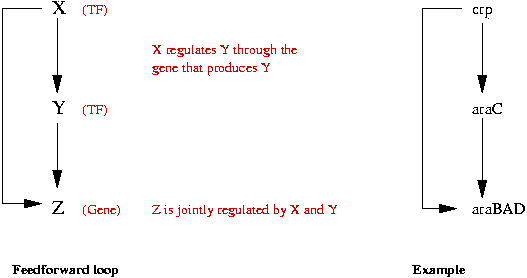• Two kinds of FF's: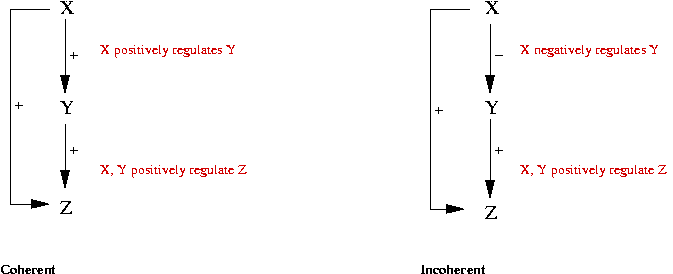• Data from E Coli:
• FF-loops occur more frequently than in random graphs.
• 85% of FF loops are coherent.
Example: Single-Input Module (SIM) motif:
• One TF regulates many genes.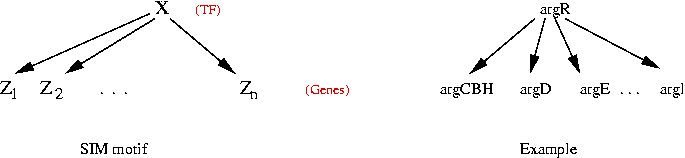• Data from E Coli:
• 24 such motifs
• Large SIM's, that occur in E Coli, are highly unlikely in random graphs.

Motif function: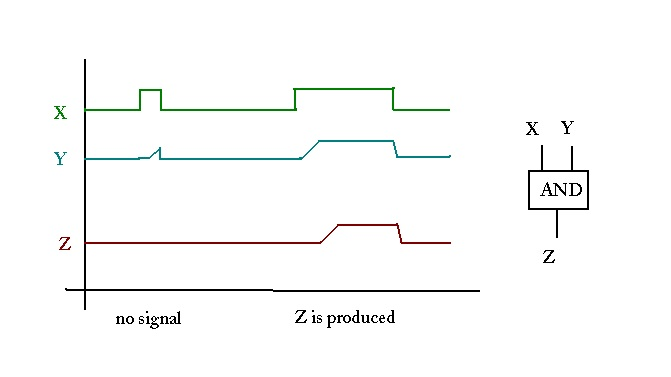Coordination:

• Coordination is needed to achieve a larger, higher-level function.

• In a computer, there is an explicit module that controls the sequence of events: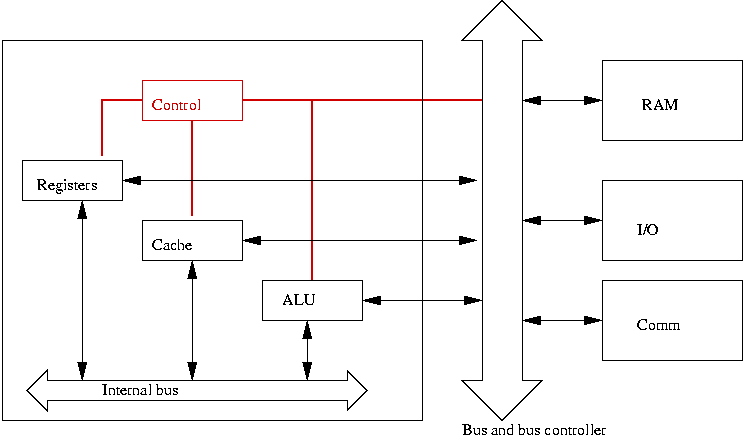• But in biological systems? Is there a central controller?

#### Network dynamics

Static properties of graphs:

• Examples: connectivity, degree distribution, cluster coefficient.

• Limitations:
• Static properties don't explain behavior.
• Static properties may not help with small networks.

Boolean networks: a model of network dynamics

Example: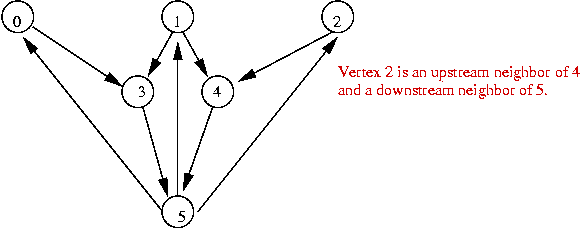• Each vertex is in one of two states:
• "On" (gene is turned on)
• "Off" (gene is turned off)

• Use 1 for "on" and 0 for "off".
• Example: suppose only node 5 is "on":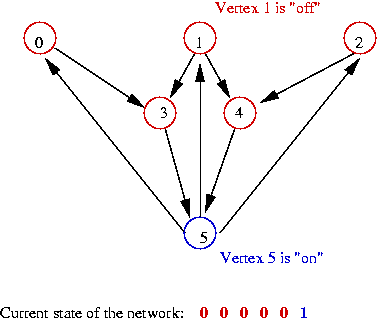• Example: nodes 0, 1, 2, 5 are "on":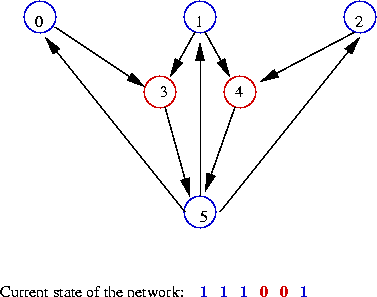• The state of the network is itself a number:
• In the first state above: state = 000001
=> binary value 1
• In the second state above: state = 111001
=> binary value 57

• The evolution of a boolean network:
• At each step: apply "rules" to current state to get next state.
• Repeat.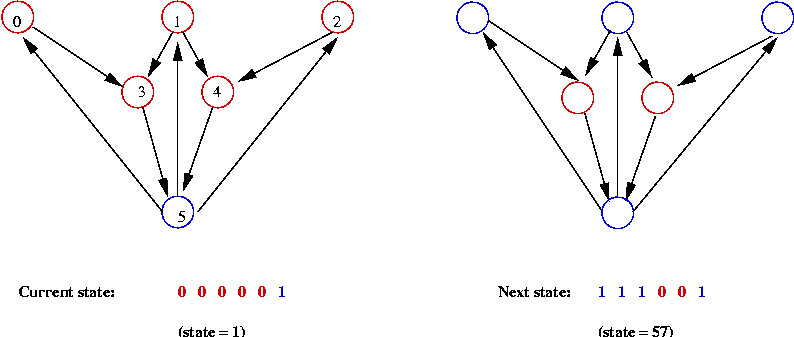• What are the "rules"?
• A rule specifies how inputs to a node affect the next state.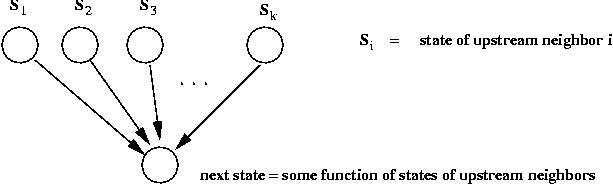• Thus, for vertex v, suppose Sv is the current state.
• Suppose v has k upstream neighbors with current states S1,...,Sk.
• The next state for v is some function
S'v = F (Sv, S1,...,Sk).

• Two types of commonly-used rules:
• Unweighted-threshold:  S'v = 1, if (S1 + ... + Sk) - t > 0. S'v = 0, if (S1 + ... + Sk) - t < 0. S'v = Sv, if (S1 + ... + Sk) - t == 0.

• Weighted-threshold: associate a weight Wi with each incoming edge  S'v = 1, if (W1 S1 + ... + Wk Sk) - t > 0. S'v = 0, if (W1 S1 + ... + Wk Sk) - t < 0. S'v = Sv, if (W1 S1 + ... + Wk Sk) - t == 0.

• Weighted-thresholds model gene down-regulation: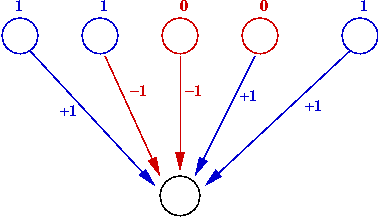• Use positive edge weight (Wi = 1) for up-regulation.
• Use negative edge weight (Wi = -11) for down-regulation.

Exercise: Consider the example from earlier:• How many possible states are there for this graph?
• Assume t = 0 and use the unweighted rules.
• Start with the state in which only vertex 5 is "on". What is the next state? And the state after that? And after that?
• Do the same for the case t = 1.

The state graph:

• Recall: each state of the network is a number.

• If there are n vertices, there are 2n possible states.

• The states are numbered States = {0, 1, ..., 2n-1}.

• Build a graph, the state graph, with vertices {0, 1, ..., 2n-1}.

• For each state S in States:
• Apply rules to compute next state S' of S.
• Place an edge from S to S' in the state graph.

Exercise: Consider this example: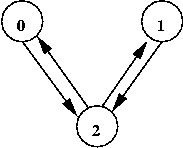• How many possible states are there for this graph?
• Assume t = 0 and use the unweighted rules.
• Compute the state graph. What do you notice?
Attractors and basins:
• In applying the rules, you can get "stuck" in a state:
=> the next state is the same.

• Such states are called attractors.

• Since there is only one outgoing edge for each state (in the state graph), each state must either go to itself or another one.

• For an attractor state S, let B(S) be the set of states that "lead" to it.
=> called the Basin of S.

Exercise: What are the attractors and basins for the above 3-vertex example?

An application to yeast cell-cycle: (Source: F.Li et al. The yeast cell-cycle is robustly designed).

• A simplified model of key proteins in the yeast cell-cycle: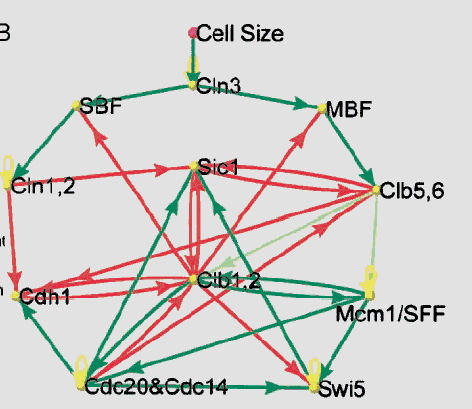• 11 vertices.
• Weighted-threshold model: t = 0.
• Green arrows: up regulation (weight = 1).
• Red arrows: down regulation (weight = -1).

• State graph has 211 = 2048 vertices.

• Part of the state graph showing a major attractor: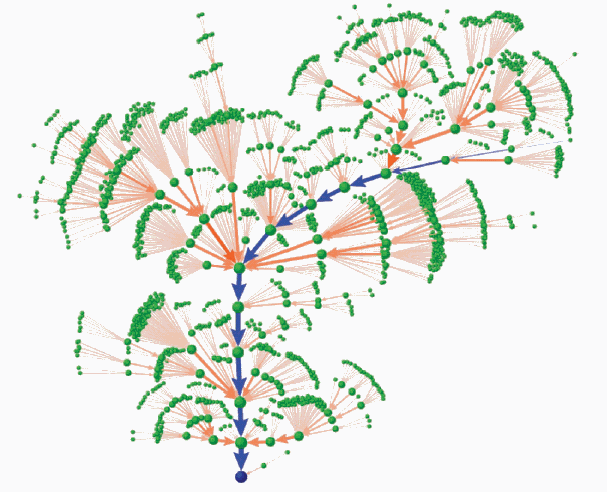• The results show that the most likely path is through a sequence of states corresponding to the cell cycle: G1, S, G2, M and back to G1.

• Significance:
• The network dynamics explain the cell cycle.
• The simple interactions of "dumb" elements in a network can create higher-level complexity.

#### Complexity

Exercise: Consider, in general, what happens for different values of the threshold t:

• What if t is too high?
• What if t is too low?